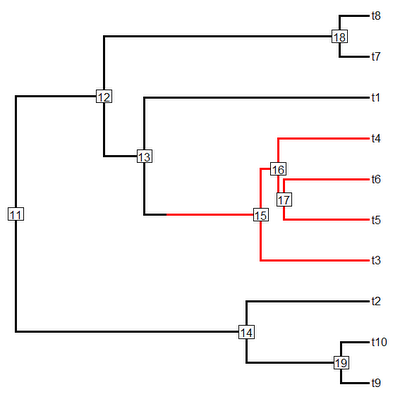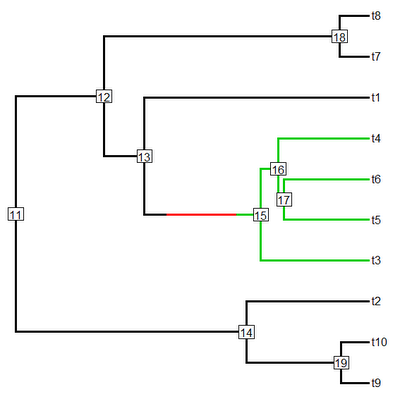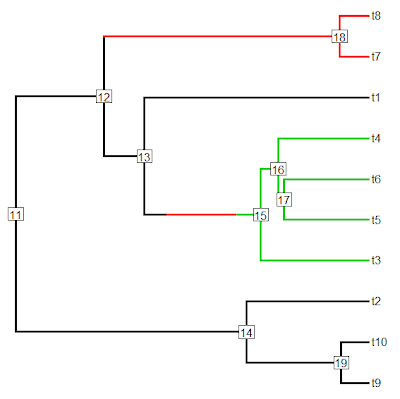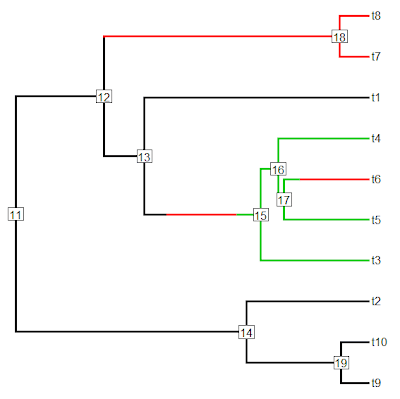## Sunday, February 12, 2012

### New version of paintSubTree

I just posted a new version of the function paintSubTree which can be used to arbitrarily paint different parts of the tree in the same format as my other functions that map discrete characters on the phylogeny (e.g., read.simmap, make.simmap, and sim.history). The reason I developed this function was mainly so that users could map arbitrary rate regimes (for instance, a specific taxonomic clade) and then use this mapping in functions such as brownie.lite and evol.vcv, but it is also handy for coloring parts of the phylogeny and then plotting these using plotSimmap.

The updated version of this function does a couple of things.

First, it is no longer necessary to provide a tree with branch lengths. Instead, if desired, users can supply a tree without branch lengths and branch lengths will be computed using the ape function compute.brlen. This function (by default) assigns the same branch lengths that one would see if visually a tree without branch lengths using plot.phylo in the ape package.

Second, this version of the function can color parts (instead of just the whole) of the stem branch for the painted sub-tree. Since TRUE and FALSE are treated as 1 & 0 during calculations in R, this change is totally backward compatible with the previous version of paintSubTree in which a logical value for the argument stem was provided.

Finally, third, the function fixes a bug that was identified by Sebastien Lavergne that came up whenever we tried to map one regime on top of a branch upon which the regime had changed. This was a book-keeping error that it was necessary to fix anyway in the process of adding the functionality of the second item, above.

Direct link to the code for the new version is here.

Let's try out this function:

> require(phytools)
> source("paintSubTree.R")
> tree<-pbtree(n=10)
> plotTree(tree,node.numbers=T,pts=F)> tree<-paintSubTree(tree,node=15,stem=0.8,state=2)
> plotSimmap(tree,node.numbers=T,pts=F,lwd=3)> tree<-paintSubTree(tree,node=15,stem=0.2,state=3)
> plotSimmap(tree,node.numbers=T,pts=F,lwd=3)> tree<-paintSubTree(tree,node=18,stem=T,state=2)
> plotSimmap(tree,node.numbers=T,pts=F,lwd=3)> tree<-paintSubTree(tree,node=match("t6",tree\$tip.label), stem=0.8,state=2)
> plotSimmap(tree,node.numbers=T,pts=F,lwd=3)Cool. Please let me know if you find any more bugs!